### 2018/05/10

WebGL 的出现，使得在浏览器环境中渲染 3D 场景变得轻而易举。但是 WebGL 和 shader 不仅可以用来渲染 3D 场景，还可以做一些其他酷酷的事情。前两天，我用 shader 技术改造 / 复刻了之前开发的一个业务页面，颇有心得和启发，不妨记录下来。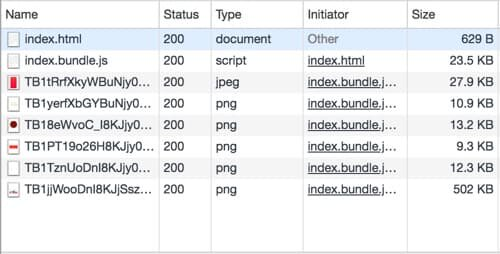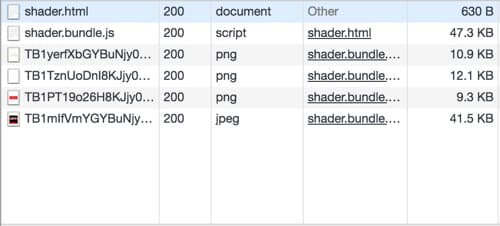# 大尺寸透明背景图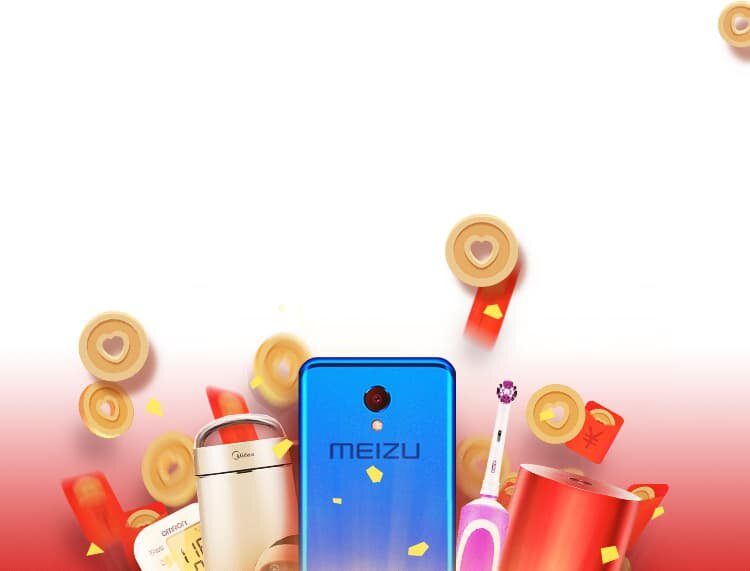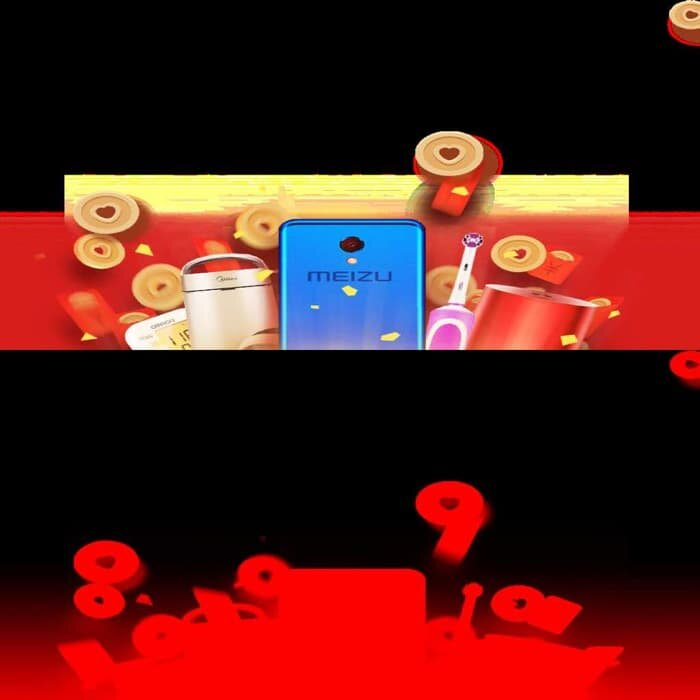``````precision mediump float;

uniform vec2 uResolution;
uniform sampler2D uImage;

void main(){

vec2 st = gl_FragCoord.xy / uResolution;

vec4 c2 = texture2D(uImage, vec2(st.x, st.y*0.5));      // 取 A​lpha 通道
vec4 c1 = texture2D(uImage, vec2(st.x, st.y*0.5+0.5));  // 取 RGB 通道

gl_FragColor = vec4(c1.xyz, c2.r > 0.6 ? c2.r : 0.0);
}``````

png 图片转化为 jpg 图片的过程，可以很轻松地在浏览器里操作 canvas 完成（示例），也可以借助一些其他的工具完成。

# 会动的背景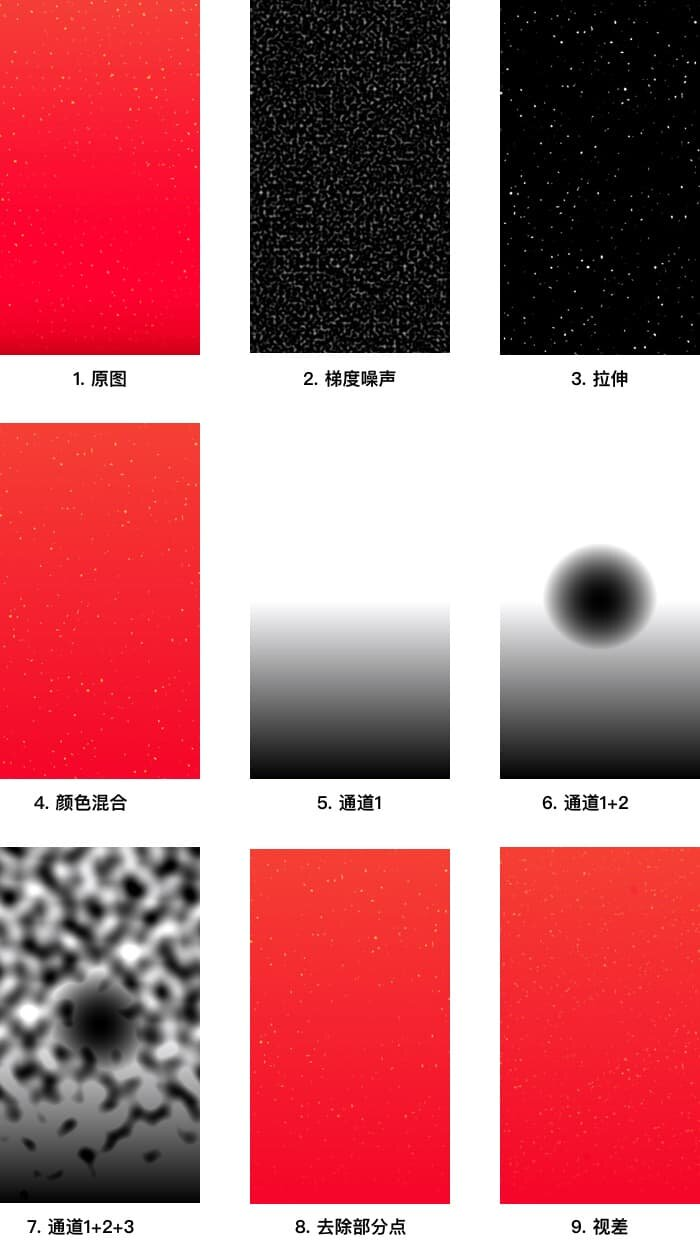``````vec2 random2(vec2 st){
st = vec2( dot(st,vec2(127.1,311.7)),
dot(st,vec2(269.5,183.3)));
st = -1.0 + 2.0*fract(sin(st)*43758.5453123);
return st;
}

float noise2(vec2 ist, vec2 fst){
vec2 g1 = random2(ist+vec2(0.0, 0.0));
vec2 g2 = random2(ist+vec2(1.0, 0.0));
vec2 g3 = random2(ist+vec2(0.0, 1.0));
vec2 g4 = random2(ist+vec2(1.0, 1.0));

vec2 f1 = fst - vec2(0.0, 0.0);
vec2 f2 = fst - vec2(1.0, 0.0);
vec2 f3 = fst - vec2(0.0, 1.0);
vec2 f4 = fst - vec2(1.0, 1.0);

float p1 = dot(g1, f1);
float p2 = dot(g2, f2);
float p3 = dot(g3, f3);
float p4 = dot(g4, f4);

fst = smoothstep(0.0, 1.0, fst);

float p = mix(
mix(p1, p2, fst.x),
mix(p3, p4, fst.x),
fst.y
);

return p;
}

float inFrag(){
vec2 st = gl_FragCoord.xy / uResolution.xx;
st = st * 60.0;
float res = noise2(floor(st), fract(st));
return res;
}

void main(){
float pct = inFrag();
gl_FragColor = vec4(vec3(pct), 1.0);
}``````

``````function initFrag(){
...
res = step(0.5, res);
return res;
}``````

``res = smoothstep(0.35, 0.5, res);``

``````vec3 bgColor(){
float y = gl_FragCoord.y / uResolution.y;
vec3 c1 = vec3(0.96, 0.02, 0.16);
vec3 c2 = vec3(0.96, 0.25, 0.21);
return mix(c1, c2, y);
}

void main(){
...

vec3 cRed = bgColor();
vec3 cYello = vec3(0.96, 0.70, 0.26);

gl_FragColor = vec4(mix(cRed, cYello, pct), 1.0);
}``````

• 原图中，页面下半部分的碎片比较透明度，越往页面下方，碎片就越透明（融入了红色背景）。
• 原图中，中间圈圈部分（即红色窗格占据的部分）没有碎片。
• 原图中，碎片的分布没有这么均匀，常有一小块区域完全没有碎片的情况，似乎有一种尺寸更大的随机变量在影响。

``````void main() {

float pct = inFrag();
pct = min(pct, yFactor());
pct = min(pct, rFactor());
pct = min(pct, mFactor());

...
}``````

``````float inFrag(){
vec2 st = gl_FragCoord.xy / uResolution.xx;
st = st * 60.0;
st.y += uTime * 2.0;  // 增加与时间相关的偏移量
float res = noise2(floor(st), fract(st));
res = smoothstep(0.35, 0.5, res);
return res;
}``````

``````void main() {

float pct = inFrag();
pct = min(pct, yFactor());
pct = min(pct, rFactor());
pct = min(pct, mFactor());

float pct2 = inFrag2();
pct2 = min(pct2, yFactor());
pct2 = min(pct2, rFactor());
pct2 = min(pct2, mFactor());

pct = max(pct, pct2);

...
}``````

# 手绘图案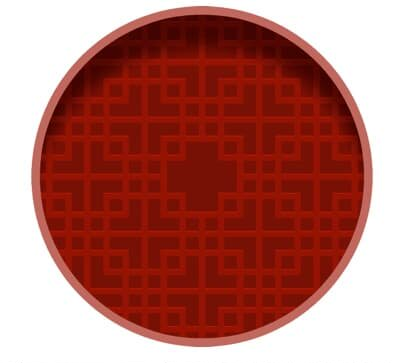``````// 绘制线的函数 veins
float line(float e, float w, float d, float p){
float e1 = e - w/2.0;
float e2 = e + w/2.0;
return smoothstep(e1 - d / 2.0, e1 + d / 2.0, p) *
smoothstep(e2 + d / 2.0, e2 - d / 2.0, p);
}

// 绘制网格
vec3 veins(){
float r = uResolution.x * 0.4;
vec2 center = vec2(uResolution.x/2.0, uResolution.y-r-5.0);
vec2 st = gl_FragCoord.xy - center;
st /= uResolution.x * 0.5;

float p = line(0.0, 0.3, 0.2, st.x);

return mix(veinsBgColor, veinsFgColor, p);
}

// 主函数
void main(){
vec3 res = veins();

gl_FragColor = vec4(vec3(res), 1.0);
}``````

main 函数调用 veins 函数，veins 又调用 line 函数得到一个灰度值，然后混合两种颜色。上述程序的结果如下图所示。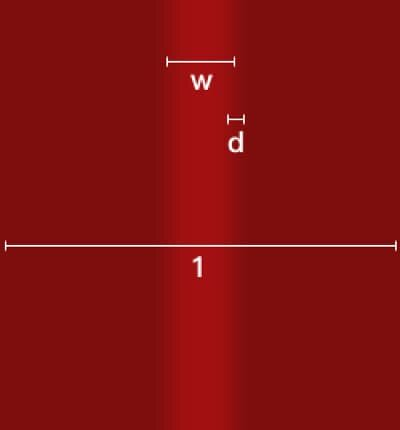``````float maxList(float list){
float res = list;
for(int i=0; i<20; i++){
if(list[i]>res){
res = list[i];
}
}
return res;
}

vec3 veins(){
...

float p = 0.0;
float pl;
pl = line(0.29, 0.035, 0.003, st.x);
pl = line(0.58, 0.035, 0.003, st.x);
...
pl = line(-0.58, 0.035, 0.003, st.y);

p = maxList(pl);

...
}``````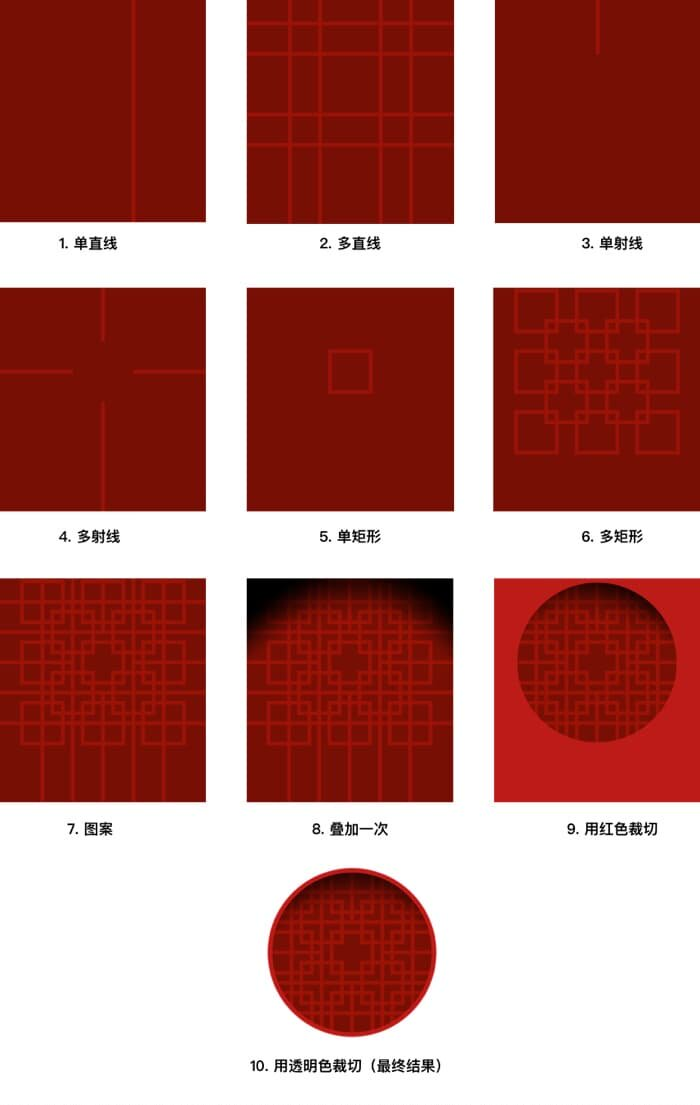``````float rayV(vec2 ep, float w, float d,  float dir, vec2 st){
float pct = line(ep.x, w, d, st.x);
if((st.y - ep.y) * dir < 0.0){
pct = 0.0;
}
return pct;
}

float rayH(vec2 ep, float w, float d,  float dir, vec2 st){
float pct = line(ep.y, w, d, st.y);
if((st.x - ep.x)* dir < 0.0){
pct = 0.0;
}
return pct;
}

float box(vec2 center, float width, float height, float w, float d, vec2 st){

float l1 = line(center.x, width+w, d, st.x);
float l2 = line(center.y, height+w, d, st.y);

float inBox = l1*l2;
float plist;

plist = line(center.x+width*0.5, w, d, st.x);
plist = line(center.x-width*0.5, w, d, st.x);
plist = line(center.y+height*0.5, w, d, st.y);
plist = line(center.y-height*0.5, w, d, st.y);

float p = maxList(plist);
p *= inBox;
return p;
}``````

``````    float p = 0.0;
float pl;
pl = line(0.29, 0.035, 0.003, st.x);
pl = line(0.58, 0.035, 0.003, st.x);
pl = line(-0.29, 0.035, 0.003, st.x);
pl = line(-0.58, 0.035, 0.003, st.x);
pl = line(0.29, 0.035, 0.003, st.y);
pl = line(0.58, 0.035, 0.003, st.y);
pl = line(-0.29, 0.035, 0.003, st.y);
pl = line(-0.58, 0.035, 0.003, st.y);

pl = rayV(vec2(0.0, 0.29), 0.035, 0.003, 1.0, st);
pl = rayV(vec2(0.0, -0.29), 0.035, 0.003, -1.0, st);
pl = rayH(vec2(0.29, 0.0), 0.035, 0.003, 1.0, st);
pl = rayH(vec2(-0.29, 0.0), 0.035, 0.003, -1.0, st);

p = maxList(pl);

float pl2;

pl2 = box(vec2(0.0, 0.0), 0.39, 0.39, 0.035, 0.003, st);

pl2 = box(vec2(0.29, 0.29), 0.39, 0.39, 0.035, 0.003, st);
pl2 = box(vec2(-0.29, 0.29), 0.39, 0.39, 0.035, 0.003, st);
pl2 = box(vec2(-0.29, -0.29), 0.39, 0.39, 0.035, 0.003, st);
pl2 = box(vec2(0.29, -0.29), 0.39, 0.39, 0.035, 0.003, st);

pl2 = box(vec2(0.58, 0.0), 0.39, 0.39, 0.035, 0.003, st);
pl2 = box(vec2(-0.58, 0.0), 0.39, 0.39, 0.035, 0.003, st);
pl2 = box(vec2(0.0, 0.58), 0.39, 0.39, 0.035, 0.003, st);
pl2 = box(vec2(0.0, -0.58), 0.39, 0.39, 0.035, 0.003, st);

pl2 = box(vec2(0.58, 0.58), 0.39, 0.39, 0.035, 0.003, st);
pl2 = box(vec2(-0.58, 0.58), 0.39, 0.39, 0.035, 0.003, st);
pl2 = box(vec2(-0.58, -0.58), 0.39, 0.39, 0.035, 0.003, st);
pl2 = box(vec2(0.58, -0.58), 0.39, 0.39, 0.035, 0.003, st);

p = max(p, maxList(pl2));``````

``````float shadow(){
float r = uResolution.x * 0.4;
vec2 center = vec2(uResolution.x/2.0, uResolution.y-r-5.0);
vec2 st = gl_FragCoord.xy - center;
st /= uResolution.x * 0.5;

return smoothstep(0.9, 0.3, st.y+0.5*st.x*st.x-0.1);
}

vec3 veins(){
}``````

``````vec3 circle(vec3 veinsColor){

float r = uResolution.x * 0.4;
vec2 center = vec2(uResolution.x/2.0, uResolution.y-r-5.0);

vec2 dxy = gl_FragCoord.xy - center;
float dist = sqrt(dxy.x*dxy.x+dxy.y*dxy.y);

float p = dist/r;
p = smoothstep(0.95, 0.96, p);

return mix(veinsColor, borderColor, p);
}

vec4 clip(vec3 color){
float r = uResolution.x * 0.4;
vec2 center = vec2(uResolution.x/2.0, uResolution.y-r-5.0);

vec2 dxy = gl_FragCoord.xy - center;
float dist = sqrt(dxy.x*dxy.x+dxy.y*dxy.y);

float p = smoothstep(1.0, 1.02, dist/r);

return vec4(color, 1.0-p);
}

void main(){
vec3 res = veins();
res = circle(res);

gl_FragColor = clip(res);
}``````

（完）